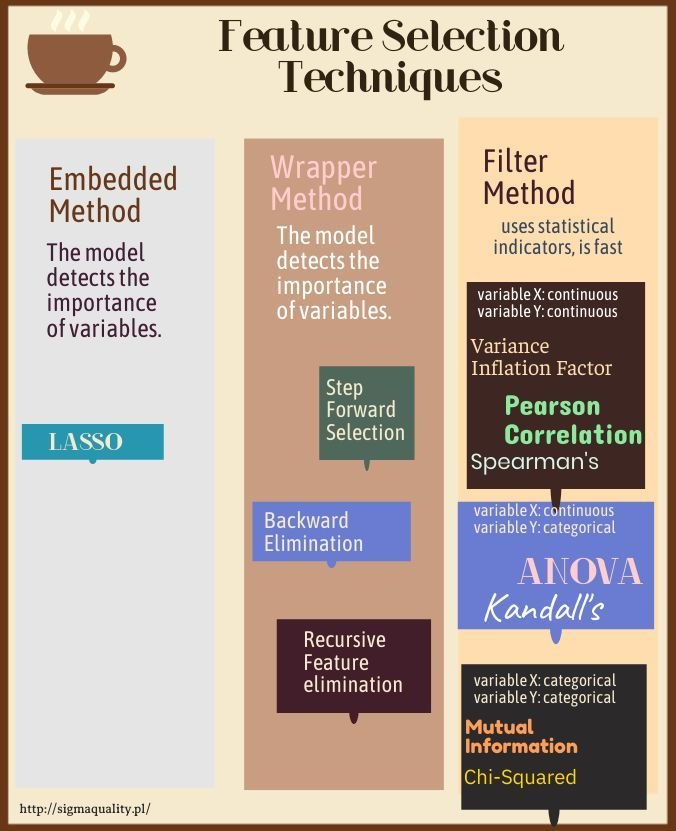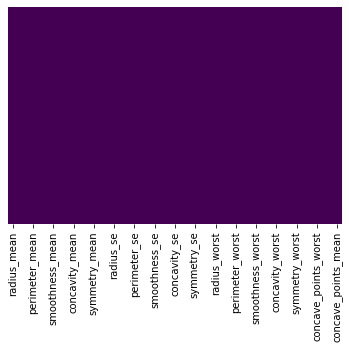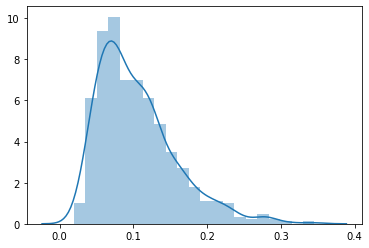# Feature Selection Techniques – Recursive Feature Elimination (RFE)

300320201719It is a greedy optimization algorithm which aims to find the best performing feature subset. It repeatedly creates models and keeps aside the best or the worst performing feature at each iteration. It constructs the next model with the left features until all the features are exhausted. It then ranks the features based on the order of their elimination.

In :
import numpy as np
import pandas as pd
import seaborn as sns
import matplotlib.pyplot as plt
from sklearn.preprocessing import LabelEncoder, OneHotEncoder
import warnings
warnings.filterwarnings("ignore")
from sklearn.model_selection import train_test_split
from sklearn.svm import SVC
from sklearn.metrics import confusion_matrix
np.random.seed(123)

In :
##  colorful prints
def black(text):
print('33[30m', text, '33[0m', sep='')
def red(text):
print('33[31m', text, '33[0m', sep='')
def green(text):
print('33[32m', text, '33[0m', sep='')
def yellow(text):
print('33[33m', text, '33[0m', sep='')
def blue(text):
print('33[34m', text, '33[0m', sep='')
def magenta(text):
print('33[35m', text, '33[0m', sep='')
def cyan(text):
print('33[36m', text, '33[0m', sep='')
def gray(text):
print('33[90m', text, '33[0m', sep='')

In :
df = pd.read_csv ('/home/wojciech/Pulpit/6/Breast_Cancer_Wisconsin.csv')
green(df.shape)

(569, 33)

Out:
id diagnosis radius_mean texture_mean perimeter_mean area_mean smoothness_mean compactness_mean concavity_mean concave points_mean texture_worst perimeter_worst area_worst smoothness_worst compactness_worst concavity_worst concave points_worst symmetry_worst fractal_dimension_worst Unnamed: 32
0 842302 M 17.99 10.38 122.8 1001.0 0.11840 0.27760 0.3001 0.14710 17.33 184.6 2019.0 0.1622 0.6656 0.7119 0.2654 0.4601 0.11890 NaN
1 842517 M 20.57 17.77 132.9 1326.0 0.08474 0.07864 0.0869 0.07017 23.41 158.8 1956.0 0.1238 0.1866 0.2416 0.1860 0.2750 0.08902 NaN
2 84300903 M 19.69 21.25 130.0 1203.0 0.10960 0.15990 0.1974 0.12790 25.53 152.5 1709.0 0.1444 0.4245 0.4504 0.2430 0.3613 0.08758 NaN

3 rows × 33 columns

### Deleting unneeded columns¶

In :
df['concave_points_worst'] = df['concave points_worst']
df['concave_points_se'] = df['concave points_se']
df['concave_points_mean'] = df['concave points_mean']

del df['Unnamed: 32']
del df['diagnosis']
del df['id']

In :
df.isnull().sum()

Out:
radius_mean                0
texture_mean               0
perimeter_mean             0
area_mean                  0
smoothness_mean            0
compactness_mean           0
concavity_mean             0
concave points_mean        0
symmetry_mean              0
fractal_dimension_mean     0
texture_se                 0
perimeter_se               0
area_se                    0
smoothness_se              0
compactness_se             0
concavity_se               0
concave points_se          0
symmetry_se                0
fractal_dimension_se       0
texture_worst              0
perimeter_worst            0
area_worst                 0
smoothness_worst           0
compactness_worst          0
concavity_worst            0
concave points_worst       0
symmetry_worst             0
fractal_dimension_worst    0
concave_points_worst       0
concave_points_se          0
concave_points_mean        0
dtype: int64
In :
import seaborn as sns

sns.heatmap(df.isnull(),yticklabels=False,cbar=False,cmap='viridis')

Out:
<matplotlib.axes._subplots.AxesSubplot at 0x7f31871d2350>### Deletes duplicates¶

there were no duplicates

In :
green(df.shape)
df.drop_duplicates(keep='first', inplace=True)
blue(df.shape)

(569, 33)
(569, 33)

In :
blue(df.dtypes)

radius_mean                float64
texture_mean               float64
perimeter_mean             float64
area_mean                  float64
smoothness_mean            float64
compactness_mean           float64
concavity_mean             float64
concave points_mean        float64
symmetry_mean              float64
fractal_dimension_mean     float64
texture_se                 float64
perimeter_se               float64
area_se                    float64
smoothness_se              float64
compactness_se             float64
concavity_se               float64
concave points_se          float64
symmetry_se                float64
fractal_dimension_se       float64
texture_worst              float64
perimeter_worst            float64
area_worst                 float64
smoothness_worst           float64
compactness_worst          float64
concavity_worst            float64
concave points_worst       float64
symmetry_worst             float64
fractal_dimension_worst    float64
concave_points_worst       float64
concave_points_se          float64
concave_points_mean        float64
dtype: object

In :
df.columns

Out:
Index(['radius_mean', 'texture_mean', 'perimeter_mean', 'area_mean',
'smoothness_mean', 'compactness_mean', 'concavity_mean',
'concave points_mean', 'symmetry_mean', 'fractal_dimension_mean',
'compactness_se', 'concavity_se', 'concave points_se', 'symmetry_se',
'perimeter_worst', 'area_worst', 'smoothness_worst',
'compactness_worst', 'concavity_worst', 'concave points_worst',
'symmetry_worst', 'fractal_dimension_worst', 'concave_points_worst',
'concave_points_se', 'concave_points_mean'],
dtype='object')

### We choose the continuous variable – compactness_mean¶

In :
print('max:',df['compactness_mean'].max())
print('min:',df['compactness_mean'].min())

sns.distplot(np.array(df['compactness_mean']))

max: 0.3454
min: 0.01938

Out:
<matplotlib.axes._subplots.AxesSubplot at 0x7f317fdf2950># Recursive Feature elimination¶

In :
X = df.drop('compactness_mean', axis=1)
y = df['compactness_mean']

from sklearn.model_selection import train_test_split

#X_train, X_test, y_train, y_test = train_test_split(X, y, test_size=0.20, random_state=123)
# Jeżeli się rzuca wtedy wycinamy stratify=y.


## I set the number of variables that will remain in the model¶

In :
Num_v = 15

In :
from sklearn.feature_selection import RFE
from sklearn.linear_model import LinearRegression

model=LinearRegression()
rfe=RFE(model,Num_v)

# Standaryzacja zmiennych

X_rfe = rfe.fit_transform(X,y)

model.fit(X_rfe,y)

print('Number of selected functions:  ',rfe.n_features_)
print()
print('The mask of selected features: ',rfe.support_)
print()
print('The feature ranking:',rfe.ranking_)
print()
print('The external estimator:',rfe.estimator_)

Number of selected functions:   15

The mask of selected features:  [False False False False  True  True  True False  True False False False
False  True  True False  True  True  True False False False False  True
True  True False False  True False  True  True]

The feature ranking: [ 3 14  4 15  1  1  1  2  1  7 11  8 16  1  1  6  1  1  1 12 17 13 18  1
1  1 10  5  1  9  1  1]

The external estimator: LinearRegression(copy_X=True, fit_intercept=True, n_jobs=None, normalize=False)


### Metoda zip na wyświetlenie rankingu cech¶

In :
PPS = rfe.ranking_

KOT_MIC = dict(zip(df, PPS))
KOT_sorted_keys_MIC = sorted(KOT_MIC, key=KOT_MIC.get, reverse=True)

for r in KOT_sorted_keys_MIC:
print (r, KOT_MIC[r])

perimeter_worst 18
perimeter_se 16
area_mean 15
texture_mean 14
texture_worst 13
fractal_dimension_se 12
concavity_worst 10
fractal_dimension_worst 9
texture_se 8
fractal_dimension_mean 7
compactness_se 6
concave points_worst 5
perimeter_mean 4
concave points_mean 2
smoothness_mean 1
compactness_mean 1
concavity_mean 1
symmetry_mean 1
area_se 1
smoothness_se 1
concavity_se 1
concave points_se 1
symmetry_se 1
area_worst 1
smoothness_worst 1
compactness_worst 1
symmetry_worst 1
concave_points_worst 1
concave_points_se 1

In :
new_cols = X.columns[rfe.support_]

In :
df2 = df[new_cols]

Out:
smoothness_mean concavity_mean concave points_mean fractal_dimension_mean smoothness_se compactness_se concave points_se symmetry_se fractal_dimension_se smoothness_worst compactness_worst concavity_worst fractal_dimension_worst concave_points_se concave_points_mean
0 0.11840 0.3001 0.14710 0.07871 0.006399 0.04904 0.01587 0.03003 0.006193 0.1622 0.6656 0.7119 0.11890 0.01587 0.14710
1 0.08474 0.0869 0.07017 0.05667 0.005225 0.01308 0.01340 0.01389 0.003532 0.1238 0.1866 0.2416 0.08902 0.01340 0.07017
2 0.10960 0.1974 0.12790 0.05999 0.006150 0.04006 0.02058 0.02250 0.004571 0.1444 0.4245 0.4504 0.08758 0.02058 0.12790

## We’re adding a result variable¶

In :
df2['compactness_mean'] = df['compactness_mean']

Out:
smoothness_mean concavity_mean concave points_mean fractal_dimension_mean smoothness_se compactness_se concave points_se symmetry_se fractal_dimension_se smoothness_worst compactness_worst concavity_worst fractal_dimension_worst concave_points_se concave_points_mean compactness_mean
0 0.11840 0.3001 0.14710 0.07871 0.006399 0.04904 0.01587 0.03003 0.006193 0.1622 0.6656 0.7119 0.11890 0.01587 0.14710 0.27760
1 0.08474 0.0869 0.07017 0.05667 0.005225 0.01308 0.01340 0.01389 0.003532 0.1238 0.1866 0.2416 0.08902 0.01340 0.07017 0.07864
2 0.10960 0.1974 0.12790 0.05999 0.006150 0.04006 0.02058 0.02250 0.004571 0.1444 0.4245 0.4504 0.08758 0.02058 0.12790 0.15990

The Backward Elimination algorithm stated that reducing variables does not improve the model. Therefore, the number of variables was left unchanged.

### OLS linear regression model for variables before reduction¶

In :
blue(df.shape)

(569, 33)

In :
X1 = df.drop('compactness_mean', axis=1)
y1 = df['compactness_mean']

In :
from statsmodels.formula.api import ols
import statsmodels.api as sm

model_fit = model.fit()

print('R2: %.6f' % model_fit.rsquared)
#blue(model_fit.summary())

R2: 0.980200


### OLS linear regression model for variables after reduction¶

In :
blue(df2.shape)

(569, 16)

In :
X2 = df2.drop('compactness_mean', axis=1)
y2 = df2['compactness_mean']

In :
from statsmodels.formula.api import ols
import statsmodels.api as sm


R2: 0.960830